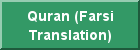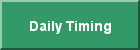بِسْمِ اللَّهِ الرَّحْمَنِ الرَّحِيمِ

In The Name of God, The All Gracious, The All Merciful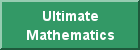You are visitor: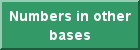Introduction to the Prime-Index Mathematics Analysis Generating the Quran

The Mathematics of Prime-Index, Generator of the Quran, (please click on the “Prime-Index Mathematic...” button) provides a mathematical basis by which the Quran is generated. Ultimate Mathematics is a 19-based blind analysis approach and it generates the “Hafs” (please click on the “Quran verse structure” button) version of the Quran in circulation, however, it only generates 127 verses for chapter 9. The analysis methodology, based on primes, twin primes, twin prime companions, composites, semi-primes, tri-primes, quad-primes, quint-primes … and their respective positional ranks or indices as well as inter-indices relations is beyond human knowledge of number theory and therefore it is a mathematical problem with no known solution.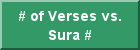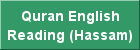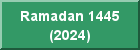25:70-- Exempted are those who repent, attain faith and lead a righteous life,
then for these, God will change their sins into credit. And God is All Forgiving, All Merciful.A few salient features of the Prime-Index Mathematics

Index             Prime          Composite
19                   67                    30
• Chapter 67 has 30 verses.
• 19 x 67 = 1273 which is the frequency of the word God from 1:1 to 9:127.
• 19 x 30 = 570 and 570th Gaussian Prime is 9127
• Chapter 9 has 127 verses.
•  570 is the frequency of the initials in Chapter 32.
• The exponent of the 19th Mersenne Prime is 4253
• Chapter 42 has 53 verses.
• The exponent of the 19th Gaussian Mersenne Prime is 353.
• 353 is the frequency of HaMeem in Chapter 42.                               Permutation Group Properties of 4253
• The 19th group element is 5234.
• The index of composite 5234  is 4537.
• Chapter 45 has 37 verses.
• The 22nd group element is 5342.
• The index of 5342 is 4635.
• Chapter 46 has 35 verses.
• The 9th group element is 3425.
• 3425 is the index of composite 3975.
• Chapter 39 has 75 verses.
• The 10th group element is 3452.
• The index of composite 3452 is 2969.
• Chapter 29 has 69 verses.
• The 19th semi-prime is 55.
• 5519 is the index of composite 6346.
• 6346 is the total number of verses in the Quran.
• 1955 is the 1657th composite.
• 1657 in base 19 is 9127 in base 10.